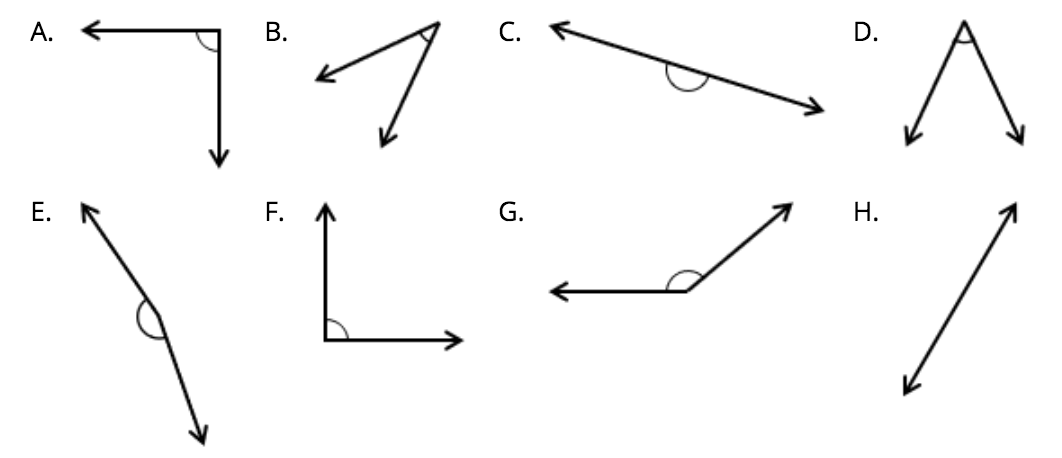# Shapes and Angles

## Objective

Identify and draw right, obtuse, and acute angles.

## Common Core Standards

### Core Standards

?

• 4.G.A.1 — Draw points, lines, line segments, rays, angles (right, acute, obtuse), and perpendicular and parallel lines. Identify these in two-dimensional figures.

?

• 3.G.A.1

## Criteria for Success

?

1. Understand that a right angle is a square corner.
2. Understand that an acute angle is one that is less than a right angle.
3. Understand that an obtuse angle is one that is greater than a right angle but less than a straight angle.
4. Understand that a straight angle is one that is formed by three points that are all on the same line.
5. Determine whether angles are right, obtuse, or acute using a right-angle template (MP.5).
6. Draw right, obtuse, and acute angles (MP.5, MP.6).

## Tips for Teachers

?

• Note that students will later see that a right angle is the angle formed by perpendicular lines and measures 90°, more precise definitions of right angles. However, because these terms and concepts have not yet been introduced, the definition is somewhat informal for the time being.
• Students are going to create a right-angle template in this lesson. Have students save this template for future lessons.

### Lesson Materials

• Template: Shapes (1 per student or small group) — See Anchor Task 1 for how to prepare this material before the lesson.
• Blank Paper (1 sheet per student) — Ideally the paper is circular, though this isn't necessary
• Template: Angles (1 per student or small group) — See Anchor Task 2 for how to prepare this material before the lesson.

#### Fishtank Plus

• Problem Set
• Student Handout Editor
• Vocabulary Package

?

### Problem 1

Sort the shapes (cut out from Template: Shapes) into groups. You may sort them any way you want and into as many groups as you want.

#### References

EngageNY Mathematics Grade 4 Mathematics > Module 4 > Topic A > Lesson 2Concept Development Problem 1

Grade 4 Mathematics > Module 4 > Topic A > Lesson 2 of the New York State Common Core Mathematics Curriculum from EngageNY and Great Minds. © 2015 Great Minds. Licensed by EngageNY of the New York State Education Department under the CC BY-NC-SA 3.0 US license. Accessed Dec. 2, 2016, 5:15 p.m..

Modified by Fishtank Learning, Inc.

### Problem 2

Determine whether each of the angles (on Template: Angles) are less than, greater than, or equal to right angles.

#### References

EngageNY Mathematics Grade 4 Mathematics > Module 4 > Topic A > Lesson 2Concept Development Problem 2

Grade 4 Mathematics > Module 4 > Topic A > Lesson 2 of the New York State Common Core Mathematics Curriculum from EngageNY and Great Minds. © 2015 Great Minds. Licensed by EngageNY of the New York State Education Department under the CC BY-NC-SA 3.0 US license. Accessed Dec. 2, 2016, 5:15 p.m..

Modified by Fishtank Learning, Inc.

### Problem 3

Draw an example of a right angle, an acute angle, an obtuse angle, and a straight angle.

#### References

EngageNY Mathematics Grade 4 Mathematics > Module 4 > Topic A > Lesson 2Concept Development Problem 3

Grade 4 Mathematics > Module 4 > Topic A > Lesson 2 of the New York State Common Core Mathematics Curriculum from EngageNY and Great Minds. © 2015 Great Minds. Licensed by EngageNY of the New York State Education Department under the CC BY-NC-SA 3.0 US license. Accessed Dec. 2, 2016, 5:15 p.m..

## Problem Set & Homework

### Discussion of Problem Set

• #1(c) and 1(f) are both right angles. Describe their position. Does the orientation of an angle determine whether it is right, acute, or obtuse?
• In #3(a), each ray shared the same endpoint. The shared endpoint is called a vertex. Label the points on your angles in Problem 3. Identify the vertex in Problems 3(b) and 3(c) with your partner.
• What is the difference between a straight angle and a line?
• Where else in your environment have you seen right angles?
• How did the right-angle template help you recognize and draw angles? How can a right-angle template help you recognize an acute angle?
• How does the right-angle template help you visualize the interior of an angle? Where would I find the interior of an angle that I’ve drawn?
• What does the exterior of an angle refer to?

?

Use the following angles to answer the questions below.Write the letters of the shapes that are:

right angles: _____________________

acute angles: _____________________

obtuse angles: _____________________

straight angles: _____________________